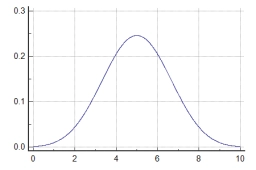# Probability 80560

I have 3 sources, and their failure probability is 0.1. Calculate the probability that:
a) none will have a malfunction
b) 1 will have a breakdown
c) at least 1 will have a fault
d) they will all have a breakdown

a =  0.729
b =  0.243
c =  0.271
d =  0.001

### Step-by-step explanation:Did you find an error or inaccuracy? Feel free to write us. Thank you!

Tips for related online calculators
Looking for a statistical calculator?
Would you like to compute the count of combinations?